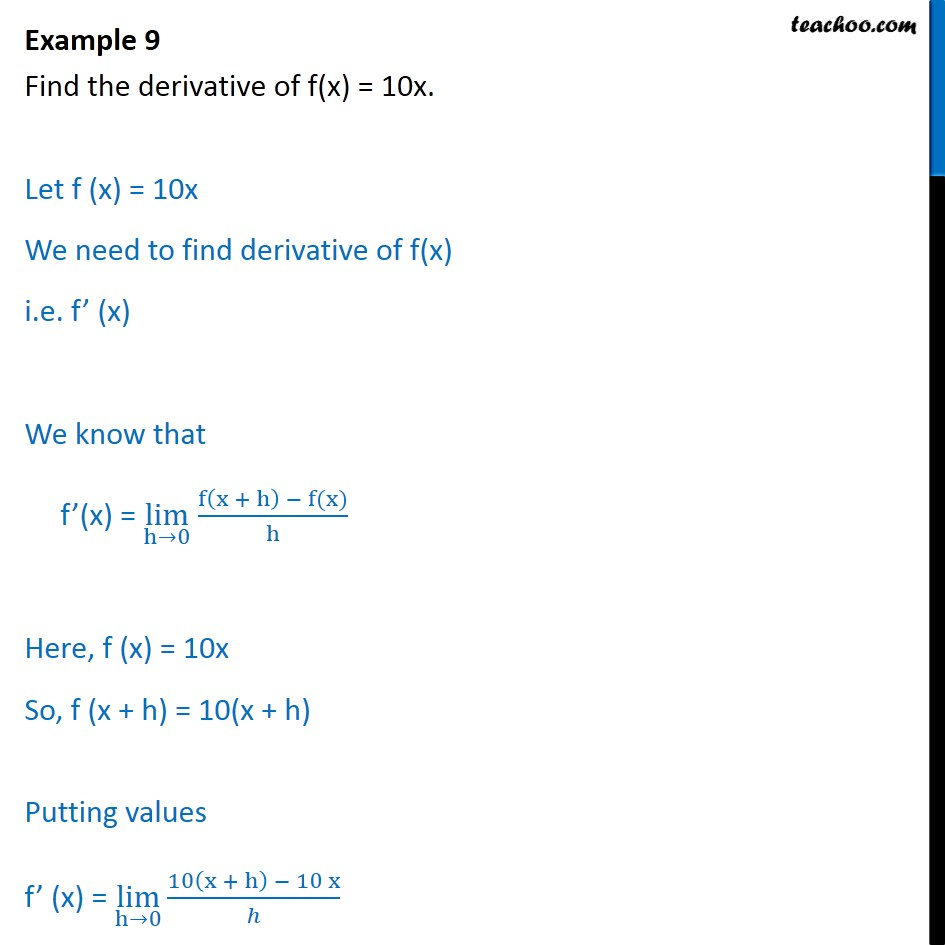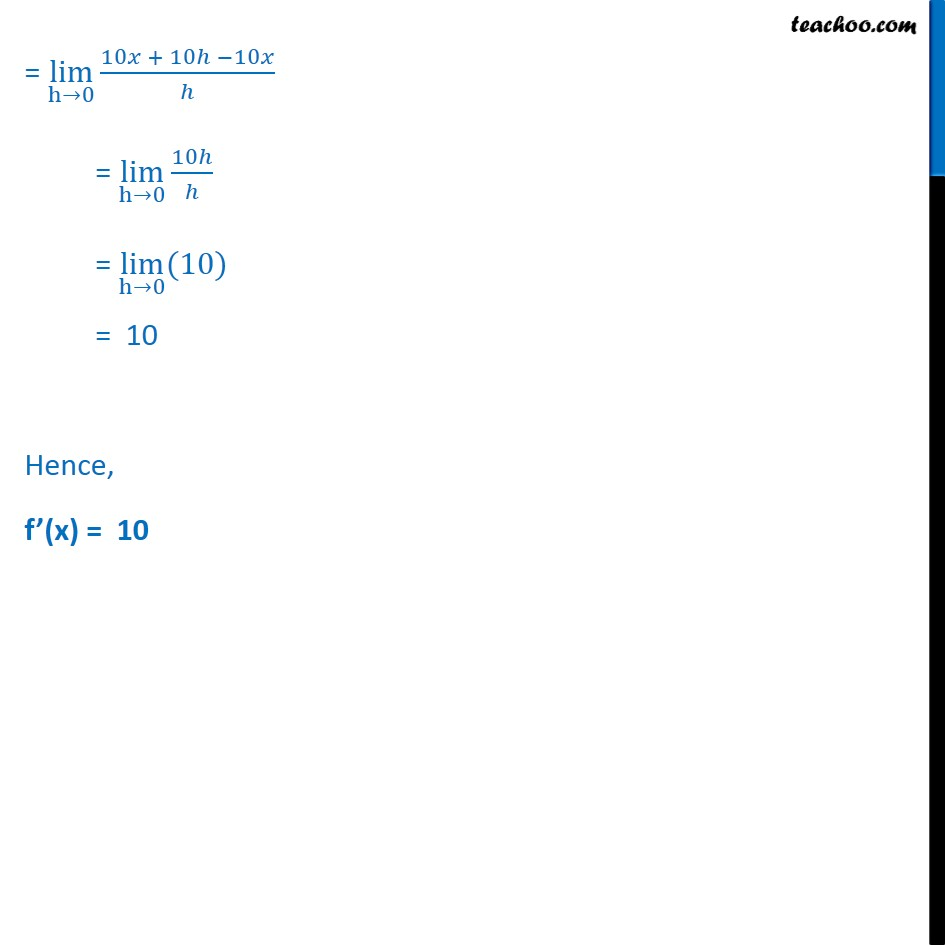Derivatives by 1st principle - At a general point

Chapter 13 Class 11 Limits and Derivatives
Concept wiseSolve all your doubts with Teachoo Black (new monthly pack available now!)

### Transcript

Example 9 Find the derivative of f(x) = 10x. Let f (x) = 10x We need to find derivative of f(x) i.e. f’ (x) We know that f’(x) = lim﷮h→0﷯ f﷮ x + h﷯ − f(x)﷯﷮h﷯ Here, f (x) = 10x So, f (x + h) = 10(x + h) Putting values f’ (x) = lim﷮h→0﷯﷮ 10 x + h﷯ − 10 x﷮ℎ﷯﷯ = lim﷮h→0﷯﷮ 10𝑥 + 10ℎ −10𝑥﷮ℎ﷯﷯ = lim﷮h→0﷯﷮ 10ℎ﷮ℎ﷯﷯ = lim﷮h→0﷯﷮(10)﷯ = 10 Hence, f’(x) = 10